Ch 1. Basics Multimedia Engineering Fluids MassDensity IdealGas Law Viscosity SurfaceTension VaporPressure
 Chapter 1. Basics 2. Fluid Statics 3. Kinematics 4. Laws (Integral) 5. Laws (Diff.) 6. Modeling/Similitude 7. Inviscid 8. Viscous 9. External Flow 10. Open-Channel Appendix Basic Math Units Basic Equations Water/Air Tables Sections Search eBooks Dynamics Fluids Math Mechanics Statics Thermodynamics Author(s): Chean Chin Ngo Kurt Gramoll ©Kurt GramollFLUID MECHANICS - CASE STUDY SOLUTION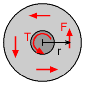Torque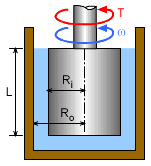Problem Diagram The liquid viscosity between the cylinder and container will resist motion. A high viscous liquid will require a high torque to keep it rotating. Thus, as expected, the viscosity of the liquid will be directly related to the torque needed to keep the cylinder rotating. First, define the toque in terms of the resisting force in the liquid. The torque is given by multiplying the force with its moment arm,      T = Fr The force can be expressed in terms of the shear stress and area as follows:      F = τ (2πrL) = T/r Rearrange terms to yield      τ = T / 2πr2L According to Newton's law of viscosity for Newtonian fluids,      τ = -μ du/dr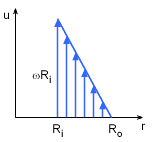Velocity Profile of the Fluid between the Container and Cylinder The negative sign is inserted to indicate that u decreases as r increases. Note that the velocity profile is assumed to be linear, as shown in the figure. The end conditions are known and are given as u = ωRi at r = Ri and u = 0 at r = Ro. Equating the above two equations and integrating from r = Ri to Ro gives,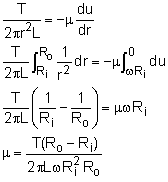For ω = 55 rev/min, T = 0.9 N-m, L = 0.3 m, Ri = 0.12m and Ro = 0.13 m, the viscosity of the liquid is calculated to be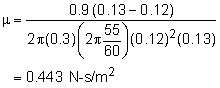If the gap between the wall and cylinder is small, then Ro - Ri => tgap, and Ri2 Ro => r3 where r is the mean radius.

Practice Homework and Test problems now available in the 'Eng Fluids' mobile app
Includes over 250 problems with complete detailed solutions.
Available now at the Google Play Store and Apple App Store.